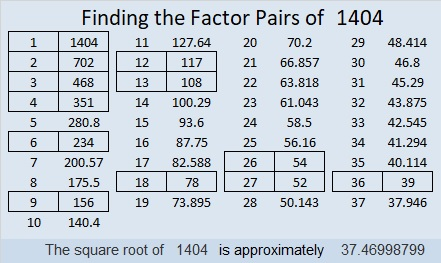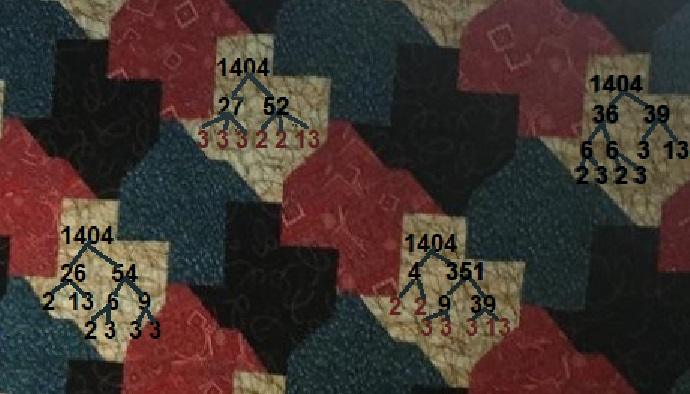# 1404 Texas Tessellation

I recently visited family members in Texas. My daughter-in-law is awesome at both mathematics and quilting. My photo does not do her work justice, but Texas is tessellated in this quilt! She also carefully chose the fabrics she pieced together. Do they remind you of anything for which Texas is famous?Someone else designed the pattern, but piecing these pieces together was not the easiest sewing project.

I wondered if anyone else had thought to tessellate Texas and found a couple of examples on twitter. As this first one asked, should we call this Texellation?

Now I’ll tell you something about the number 1404:

• 1404 is a composite number.
• Prime factorization: 1404 = 2 × 2 × 3 × 3 × 3 × 13, which can be written 1404 = 2² × 3³ × 13.
• 1404 has at least one exponent greater than 1 in its prime factorization so √1404 can be simplified. Taking the factor pair from the factor pair table below with the largest square number factor, we get √1404 = (√36)(√39) = 6√39.
• The exponents in the prime factorization are 2, 3, and 1. Adding one to each exponent and multiplying we get (2 + 1)(3 + 1)(1 + 1) = 3 × 4 × 2 = 24. Therefore 1404 has exactly 24 factors.
• The factors of 1404 are outlined with their factor pair partners in the graphic below.1404 is the hypotenuse of a Pythagorean triple:
540-1296-1404 which is (5-12-13) times 108

Since 1404 has so many factors, it also has MANY different factor trees. Here are four of them mixed in with some Texas tessellations!This site uses Akismet to reduce spam. Learn how your comment data is processed.